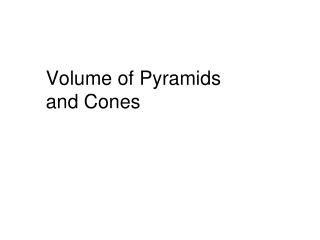# Volume of Pyramids and Cones - PowerPoint PPT PresentationDownload PresentationVolume of Pyramids and Cones

Volume of Pyramids and ConesDownload Presentation## Volume of Pyramids and Cones

- - - - - - - - - - - - - - - - - - - - - - - - - - - E N D - - - - - - - - - - - - - - - - - - - - - - - - - - -
##### Presentation Transcript

1. Volume of Pyramids and Cones

2. Finding Volumes of Pyramids and Cones * In Lesson 6.4, you learned that the volume of a prism is equal to Bh, where B is the area of the base, and h is the height. From the figure at the left, it is clear that the volume of the pyramid with the same base area B and the same height h must be less than the volume of the prism. The volume of the pyramid is one third the volume of the prism.

3. Theorems: * Volume of a Pyramid – The volume V of a pyramid is V = Bh, where B is the area of the base and h is the height.

4. Theorems: * Volume of a Cone – The volume of a cone is V = Bh = r2h, where B is the area of the base, h is the height and r is the radius of the base.

5. Ex. 2: Finding the volume of a cone Find the volume of each cone.

6. Ex. 2: Finding the volume of a cone Find the volume of each cone.

7. Ex. 3: Using the Volume of a Cone

8. Ex. 1: Finding the volume of a pyramid * Find the volume of the pyramid with the regular base.

9. Ex. 1: Finding the volume of a pyramid * The base can be divided into six equilateral triangles.

10. Ex. 1: Finding the volume of a pyramid

11. EXTRA CREDIT * Nautical prisms. A nautical prism is a solid piece of glass as shown. Find its volume.

12. EXTRA CREDIT * Automobiles. If oil is being poured into the funnel at a rate of 147 milliliters per second and flows out of the funnel at a rate of 42 milliliters per second, estimate the time it will take for the funnel to overflow. (1 mL = 1 cm3).

13. Ex. 5: Using the volume of a cone The rate of accumulation of oil in the funnel is 147 – 42 = 105 mL/s. To find the time it will take for the oil to fill the funnel, divide the volume of the funnel by the rate of accumulation of oil in the funnel as follows:

14. Volume and Surface Area of a Sphere

15. Surface Area of a Sphere • The surface area of a sphere is equal to: S.A =

16. Volume of a Sphere • The volume of a sphere is equal to: V =